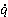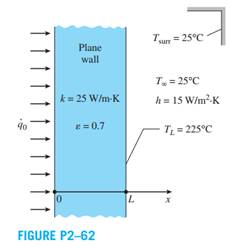Create an Account

Home / Questions / A large plane wall has a thickness L 50 cm and thermal conductivity k 25 WmK On the left surface

A large plane wall has a thickness L 50 cm and thermal conductivity k 25 WmK On the left surface

A large plane wall has a thickness L = 50 cm and thermal conductivity k = 25 W/m∙K. On the left surface (x = 0), it is subjected to a uniform heat flux0 while the surface temperature T0 is constant. On the right surface, it experiences convection and radiation heat transfer while the surface temperature is TL = 225°C and the surrounding temperature is 25°C. The emissivity and the convection heat transfer coefficient on the right surface are 0.7 and 15 W/m2 ∙K, respectively. Show that the variation of temperature in the wall can be expressed as T(x) = (0 /k) (L - x) + TL, where0 = 5130 W/m2, and determine the temperature of the left surface of the wall at x = 0.Jul 21 2020 View more View LessSubscribe To Get Solution